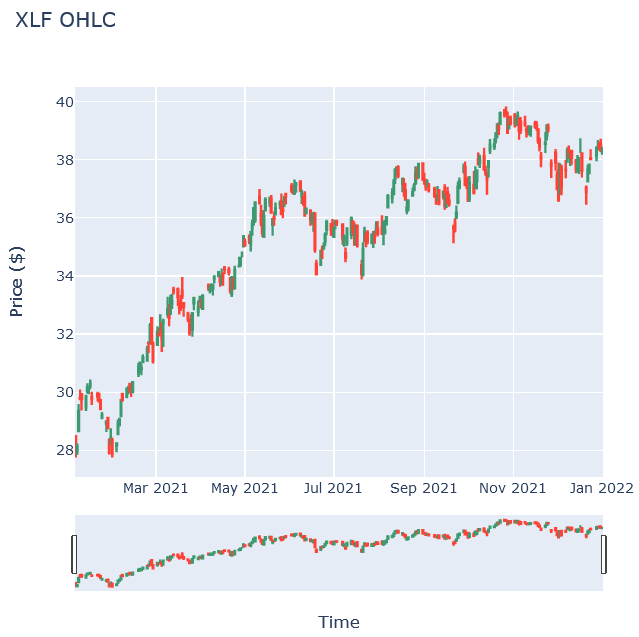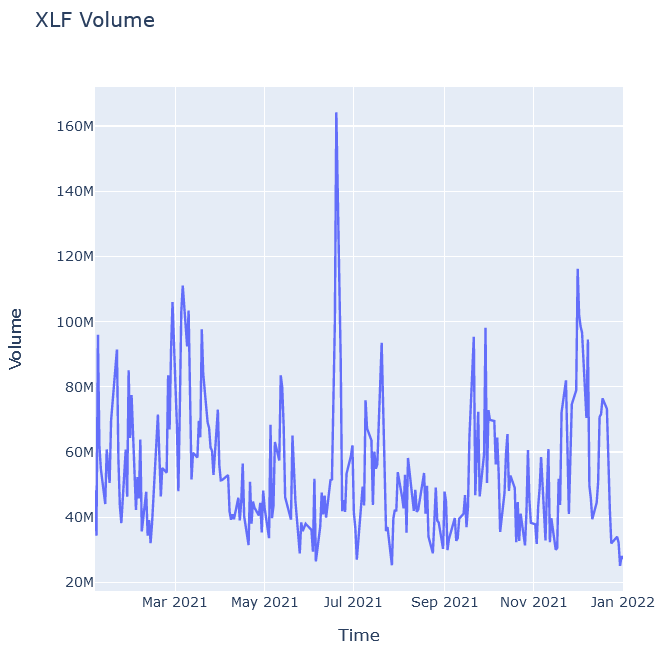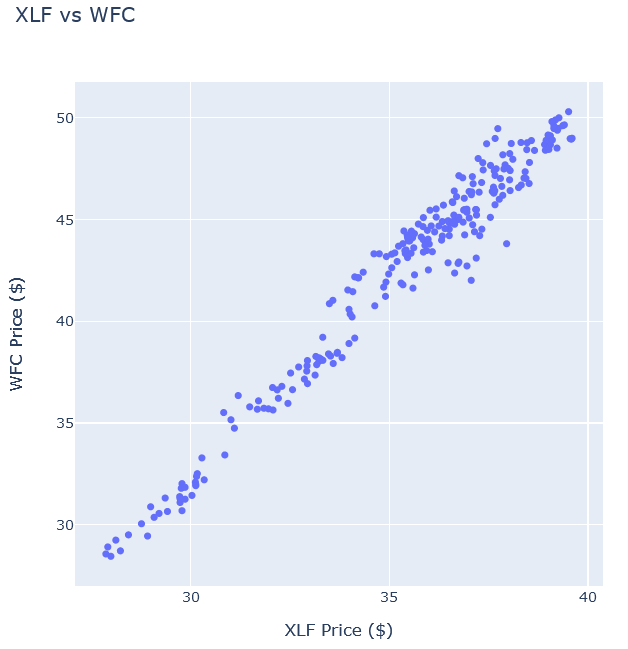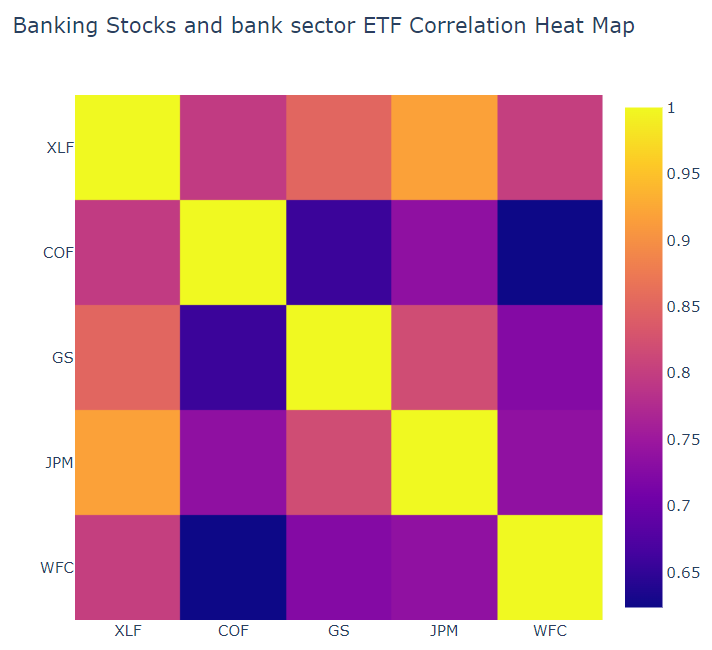# Charting

## Plotly NET

### Introduction

Plotly.NET provides functions for generating and rendering plotly.js charts in .NET programming languages. Our .NET interactive notebooks support its C# implementation.

### Import Libraries

Follow these steps to import the libraries that you need:

1. Load the assembly files and data types in their own cell.
2. #load "../Initialize.csx"
3. Load the necessary assembly files.
4. #load "../QuantConnect.csx"
#r "../Plotly.NET.dll"
#r "../Plotly.NET.Interactive.dll"
5. Import the QuantConnect, Plotly.NET, and Accord packages.
6. using QuantConnect;
using QuantConnect.Research;

using Plotly.NET;
using Plotly.NET.Interactive;
using Plotly.NET.LayoutObjects;

using Accord.Math;
using Accord.Statistics;

### Get Historical Data

Get some historical market data to produce the plots. For example, to get data for a bank sector ETF and some banking companies over 2021, run:

var qb = new QuantBook();
var tickers = new[]
{
"XLF",  // Financial Select Sector SPDR Fund
"COF",  // Capital One Financial Corporation
"GS",   // Goldman Sachs Group, Inc.
"JPM",  // J P Morgan Chase & Co
"WFC"   // Wells Fargo & Company
};
var symbols = tickers.Select(ticker => qb.AddEquity(ticker, Resolution.Daily).Symbol);
var history = qb.History(symbols, new DateTime(2021, 1, 1), new DateTime(2022, 1, 1));

### Create Candlestick Chart

You must import the plotting libraries and get some historical data to create candlestick charts.

In this example, you create a candlestick chart that shows the open, high, low, and close prices of one of the banking securities. Follow these steps to create the candlestick chart:

1. Select a Symbol.
2. var symbol = symbols.First();
3. Call the Chart2D.Chart.Candlestick constructor with the time and open, high, low, and close price IEnumerable.
4. var bars = history.Select(slice => slice.Bars[symbol]);
var chart = Chart2D.Chart.Candlestick<decimal, decimal, decimal, decimal, DateTime, string>(
bars.Select(x => x.Open),
bars.Select(x => x.High),
bars.Select(x => x.Low),
bars.Select(x => x.Close),
bars.Select(x => x.EndTime)
);
5. Call the Layout constructor and set the title, xaxis, and yaxis properties as the title and axes label objects.
6. LinearAxis xAxis = new LinearAxis();
xAxis.SetValue("title", "Time");
LinearAxis yAxis = new LinearAxis();
yAxis.SetValue("title", "Price ($)"); Title title = Title.init($"{symbol} OHLC");

Layout layout = new Layout();
layout.SetValue("xaxis", xAxis);
layout.SetValue("yaxis", yAxis);
layout.SetValue("title", title);
7. Assign the Layout to the chart.
8. chart.WithLayout(layout);
9. Show the plot.
10. HTML(GenericChart.toChartHTML(chart));

The Jupyter Notebook displays the candlestick chart.### Create Line Chart

You must import the plotting libraries and get some historical data to create line charts.

In this example, you create a line chart that shows the volume of a security. Follow these steps to create the chart:

1. Select a Symbol.
2. var symbol = symbols.First();
3. Call the Chart2D.Chart.Line constructor with the timestamps and volumes.
4. var bars = history.Select(slice => slice.Bars[symbol]);
var chart = Chart2D.Chart.Line<DateTime, decimal, string>(
bars.Select(x => x.EndTime),
bars.Select(x => x.Volume)
);
5. Create a Layout.
6. LinearAxis xAxis = new LinearAxis();
xAxis.SetValue("title", "Time");
LinearAxis yAxis = new LinearAxis();
yAxis.SetValue("title", "Volume");
Title title = Title.init($"{symbol} Volume"); Layout layout = new Layout(); layout.SetValue("xaxis", xAxis); layout.SetValue("yaxis", yAxis); layout.SetValue("title", title); 7. Assign the Layout to the chart. 8. chart.WithLayout(layout); 9. Show the plot. 10. HTML(GenericChart.toChartHTML(chart)); The Jupyter Notebook displays the line chart.### Create Scatter Plot You must import the plotting libraries and get some historical data to create scatter plots. In this example, you create a scatter plot that shows the relationship between the daily price of two securities. Follow these steps to create the scatter plot: 1. Select two Symbol objects. 2. var symbol1 = symbols.First(); var symbol2 = symbols.Last(); 3. Call the Chart2D.Chart.Point constructor with the closing prices of both securities. 4. var chart = Chart2D.Chart.Point<decimal, decimal, string>( history.Select(slice => slice.Bars[symbol1].Close), history.Select(slice => slice.Bars[symbol2].Close) ); 5. Create a Layout. 6. LinearAxis xAxis = new LinearAxis(); xAxis.SetValue("title",$"{symbol1} Price ($)"); LinearAxis yAxis = new LinearAxis(); yAxis.SetValue("title",$"{symbol2} Price ($)"); Title title = Title.init($"{symbol1} vs {symbol2}");

Layout layout = new Layout();
layout.SetValue("xaxis", xAxis);
layout.SetValue("yaxis", yAxis);
layout.SetValue("title", title);
7. Assign the Layout to the chart.
8. chart.WithLayout(layout);
9. Show the plot.
10. HTML(GenericChart.toChartHTML(chart));

The Jupyter Notebook displays the scatter plot.### Create Heat Map

You must import the plotting libraries and get some historical data to create heat maps.

In this example, you create a heat map that shows the correlation between the daily returns of the banking securities. Follow these steps to create the heat map:

1. Compute the daily returns.
2. var data = history.SelectMany(x => x.Bars.Values)
.GroupBy(x => x.Symbol)
.Select(x =>
{
var prices = x.Select(x => (double)x.Close).ToArray();
return Enumerable.Range(0, prices.Length - 1)
.Select(i => prices[i+1] / prices[i] - 1).ToArray();
}).ToArray().Transpose();
3. Call the Measures.Correlation method.
4. var corrMatrix = Measures.Correlation(data).Select(x => x.ToList()).ToList();
5. Call the Plotly.NET.Chart2D.Chart.Heatmap constructor with the correlation matrix.
6. var X = Enumerable.Range(0, tickers.Length).ToList();

var heatmap = Plotly.NET.Chart2D.Chart.Heatmap<IEnumerable<double>, double, int, int, string>(
zData: corr,
X: X,
Y: X,
ShowScale: true,
ReverseYAxis: true
);
7. Create a Layout.
8. var axis = new LinearAxis();
axis.SetValue("tickvals", X);
axis.SetValue("ticktext", tickers);

var layout = new Layout();
layout.SetValue("xaxis", axis);
layout.SetValue("yaxis", axis);
layout.SetValue("title", Title.init("Banking Stocks and bank sector ETF Correlation Heat Map"));
9. Assign the Layout to the chart.
10. heatmap.WithLayout(layout);
11. Show the plot.
12. HTML(GenericChart.toChartHTML(heatmap))

The Jupyter Notebook displays the heat map.You can also see our Videos. You can also get in touch with us via Discord.